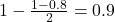## We’ll assume that the stem diameter is normally distributed. An agronomist measured stem diameter in 8 randomly selected plants of a particu

Question

We’ll assume that the stem diameter is normally distributed. An agronomist measured stem diameter in 8 randomly selected plants of a particular cultivar of wheat. The mean of the sample is 2.275 and the standard deviation is 0.238.Construct a 80% confidence interval for the population mean.

in progress 0
3 months 2021-07-19T16:29:08+00:00 1 Answers 0 views 0

The 80% confidence interval for the population mean is (2.156, 2.394).

Step-by-step explanation:

We have the standard deviation for the sample, which means that the t-distribution is used to solve this question.

The first step to solve this problem is finding how many degrees of freedom, we have. This is the sample size subtracted by 1. So

df = 8 – 1 = 7

80% confidence interval

Now, we have to find a value of T, which is found looking at the t table, with 7 degrees of freedom(y-axis) and a confidence level of. So we have T = 1.415

The margin of error is:In which s is the standard deviation of the sample and n is the size of the sample.

The lower end of the interval is the sample mean subtracted by M. So it is 2.275 – 0.119 = 2.156

The upper end of the interval is the sample mean added to M. So it is 2.275 + 0.119 = 2.394

The 80% confidence interval for the population mean is (2.156, 2.394).